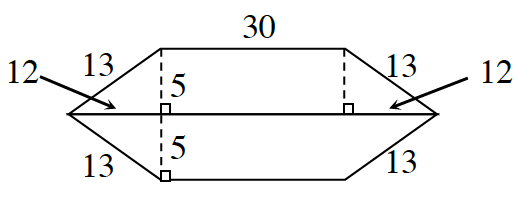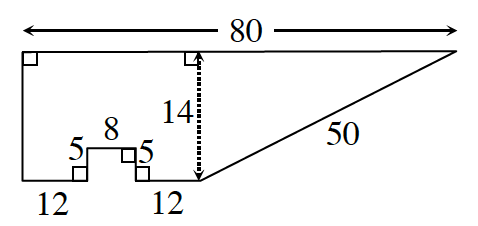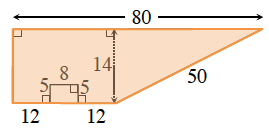### Home > CCAA > Chapter 8 Unit 7 > Lesson CC2: 8.2.1 > Problem8-38

8-38.

Find the area and perimeter of each shape below. Show your steps and work. Note: Diagrams are not drawn to scale.

1.Perimeter: Add the lengths of each of the sides around the outside of the figure.

$13+30+13+13+30+13=112$ units

The shape consists of two trapezoids of equal size. Find the area of one trapezoid and double it to find the area of the entire shape.

The area of a trapezoid can be found with the following formula:

$A=\frac{1}{2}(\text{base}_1+\text{base}_2)(\text{height})$

$420$ square units

1.You can use the equation for the area of a trapezoid to find the total area.

The trapezoid is highlighted.
Notice that there is a rectangular section missing from the trapezoid.
You will need to find that area as well.$744$ sq. units.